# Transformations of Continuous-Time Signals

## Continuous time signal:  Time is a continuous variable; the signal itself need not be continuous.

### Time Reversal

We reverse a signal x(t) by flipping it over the vertical-axis to form a new signal   y(t) = x(-t).

Example: Find

y(t) = x(-t)

where x(t) is: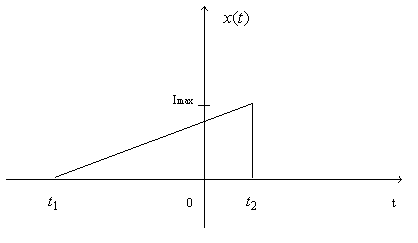### Time Scaling

The signal y(t) = x(at) is a time-scaled version of x(t).

If |a| > 1, we are SPEEDING UP x(t) by a factor of a.

If |a| < 1, we are SLOWING DOWN x(t) by a factor of a.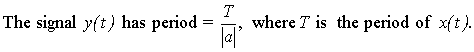Example: Given x(t), find y(t) = x(2t). This SPEEDS UP x(t)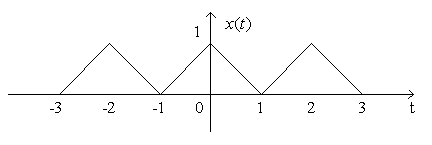What happens to the period T?

The period of x(t) is 2 and the period of y(t) is 1, because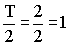Example: Given x(t), find z(t) = x(t/2). This SLOWS DOWN x(t)Example: Given y(t), find w(t) = y(3t) and v(t) = y(t/3).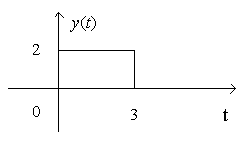### Time Shifting

y(t) = x(t - t0)

Here, the original signal x(t) is shifted by an amount t0 .

Rule: set t - t0=0 and move the origin of x(t) to t0.

Given x(t) = u(t+2) - u(t-2), find x(t-t0) and x(t+t0).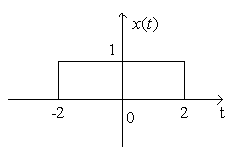Example: Determine

x(t) + x(2-t)

where x(t) = u(t+1)- u(t-2)

Step 1: which of these functions is x(t)?   A, B, C or D

Step 2: Find x(2-t)

Method I to find x(2-t): Reverse in time, then delay.

Let y(t) = x(-t)

And y(t-2) = x(-(t-2)) = x(2-t)

Method II to find x(2-t): Advance, then reverse in time.

Let w(t) = x(t +2)

As the last step, add up x(t)and x(2 - t)

Combinations of Scale and Shift

Find x(2t+1) where x(t) is: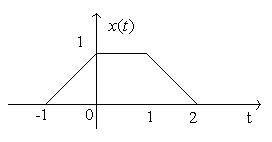Method 1: Shift then scale: x(at+b):(i) v(t)=x(t+b); (ii) y(t) =v(at)= x(at+b).

 v(t) = x(t+1) y(t) = v(2t)

Method 2: Scale then shift: x(at + b) = x(a (t + b/a)) :

 ( i ) w(t) = x(at) ; ( ii ) y(t) = w(t + b/a) = x(at+b)

 w(t) = x(2t) y(t) = w(t+1/2))  =  x(2(t+1/2 ))  =  x(2t+1)

### Amplitude Operations (Scaling or adding signals)

Example: Given x1(t), find -x1(t), 2x1(t), and .5x1(t)

Example: Given x2(t), find 1 - x2(t). This is a case of adding together two signals.

Addition of two signals: x1(t) + x2(t)

To add two signals together, we simply add the values of the signals point-by-point.

Multiplication of two signals:x2(t)u(t)

To multiply two signals together, we simply multiply the values of the signals point-by-point.